# Folded square

ABCD is a square. The square is folded on the midpoint of AB, and A is folded onto the fold, creating a shaded region. The perimeter of the shaded figure is 75. Find the area of square ABCD

S =  900

### Step-by-step explanation: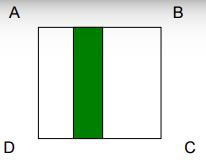Did you find an error or inaccuracy? Feel free to write us. Thank you!#### You need to know the following knowledge to solve this word math problem:

We encourage you to watch this tutorial video on this math problem:

## Related math problems and questions:

• Three shapes1/5 of a circle is shaded. The ratio of area if square to the sum of area of rectangle and that of the circle is 1:2. 60% of the square is shaded and 1/3 of the rectangle is shaded. What is the ratio of the area of circle to that of the rectangle?
• Perimeter and legsDetermine the perimeter of a right triangle if the length of one leg is 75% length of the second leg and its content area is 24 cm2.
• Rain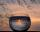How many mm of water rained the roof space 75 m2 if the empty barrel with a radius of 8 dm and height 1.2 m filled to 75% its capacity? :-)
• Rectangle - sides ratioCalculate the area of a rectangle whose sides are in ratio 3:13 and perimeter is 673.
• Percentage of waste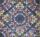In a square plate with side 75 cm we cut 4 same circles. Calculate the percentage of waste.
• A trapezoidA trapezoid 75 ft wide on top 85 ft on the bottom, the height is 120 ft. What is its area in the square yds?
• Triangle SAAThe triangle has one side long 71 m and its two internal angles is 60°. Calculate the perimeter and area of the triangle.
• SquarePoints A[9,9] and B[-4,1] are adjacent vertices of the square ABCD. Calculate the area of the square ABCD.
• One halfOne half of 2 75 is: ...
• Cross five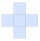The figure on the picture is composed of the same squares and has a content of 45cm². What's its perimeter?
• ISO Triangle V2The perimeter of RR triangle (isosceles) is 474 m, and the base is 48 m longer than the arms. Calculate the area of this triangle.
• Triangular prismThe base of the perpendicular triangular prism is a rectangular triangle with a hypotenuse of 10 cm and one leg of 8 cm. The prism height is 75% of the perimeter of the base. Calculate the volume and surface of the prism.
• Parallelogram ABCDWe have the parallelogram ABCD, where AB is 6.2 cm BC is 5.4 cm AC is 4.8 cm calculate the height on the AB side and the angle DAB
• ABCD squareIn the ABCD square, the X point lies on the diagonal AC. The length of the XC is three times the length of the AX segment. Point S is the center of the AB side. The length of the AB side is 1 cm. What is the length of the XS segment?
• Trapezoid ABCD v2Trapezoid ABCD has a length of bases in ratio 3:10. The area of triangle ACD is 825 dm2. What is the area of trapezoid ABCD?
• DescribedCalculate perimeter of the circle described by a triangle with sides 478, 255, 352.
• Two lands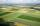The common area of the two neighboring lands is 964 m2. The second land is 77 m2 smaller than twice the size of the first land. Find the areas of each land.